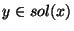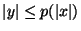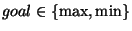Next: Terminology for graph problems Up: Introduction Previous: Introduction   Index

### NPO problems: definitions and preliminaries

The basic ingredients of an optimization problem are the set of instances or input objects, the set of feasible solutions or output objects associated with any instance, and the measure defined for any feasible solution. On the analogy of the theory of NP-completeness, we are interested in studying a class of optimization problems whose feasible solutions are short and easy-to-recognize. To this aim, suitable constraints have to be introduced. We thus give the following definition.

Definition 1

An NP optimization problem A is a fourtuple (I,sol,m,goal) such that

1.
I is the set of the instances of A and it is recognizable in polynomial time.

2.
Given an instance x of I, sol(x) denotes the set of feasible solutions of x. These solutions are short, that is, a polynomial p exists such that, for any,. Moreover, it is decidable in polynomial time whether, for any x and for any y such that,.

3.
Given an instance x and a feasible solution y of x, m(x,y) denotes the positive integer measure of y. The function m is computable in polynomial time and is also called the objective function.

4..

The class NPO is the set of all NP optimization problems.

The goal of an NPO problem with respect to an instance x is to find an optimum solution, that is, a feasible solution y such thatIn the following, opt will denote the function mapping an instance x to the measure of an optimum solution.

An NPO problem is said to be polynomially bounded if a polynomial q exists such that, for any instance x and for any solution y of x,. The class NPO PB is the set of polynomially bounded NPO problems.Next: Terminology for graph problems Up: Introduction Previous: Introduction   Index
Viggo Kann
2000-03-20